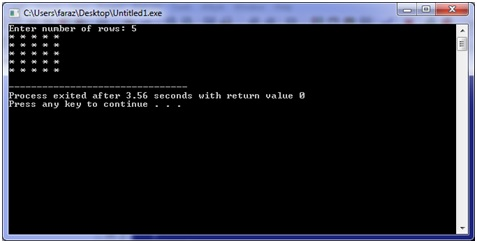# C program for printing 'Square' pattern

In this article, we are implementing logic to print Square patter using asterisks and loops (nested loops).
Submitted by Shamikh Faraz, on February 16, 2018

In this program, we will learn, how we can print square using ‘asterisks’ and ‘loops’.

C program

﻿

```#include <stdio.h>

int main()
{
int a, b, n;

printf("Enter number of rows: ");
scanf("%d",&n);

for(a=1; a<=n; ++a)
{
for(b=1; b<=n; ++b)
{
printf("* ");
}
printf("\n");
}
return 0;
}
```

Output